Скачать презентацию Chapter 6 The Second Law of Thermodynamics Study

45fad3a107b7e57377c99e7171ec5364.ppt

• Количество слайдов: 31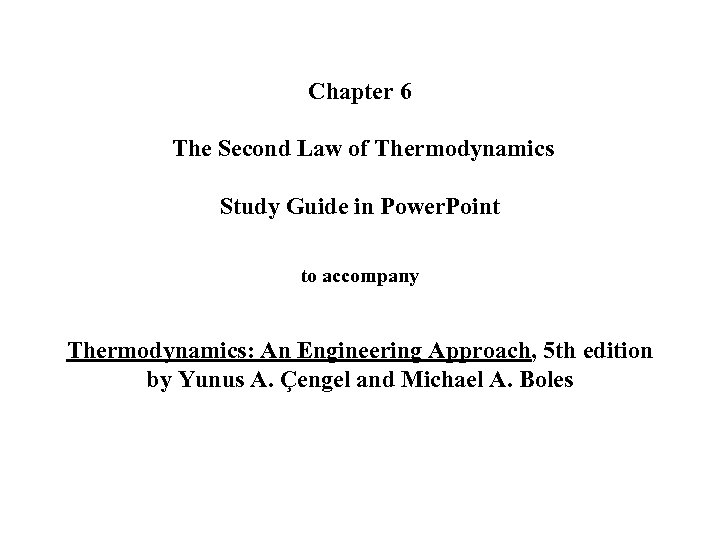Chapter 6 The Second Law of Thermodynamics Study Guide in Power. Point to accompany Thermodynamics: An Engineering Approach, 5 th edition by Yunus A. Çengel and Michael A. Boles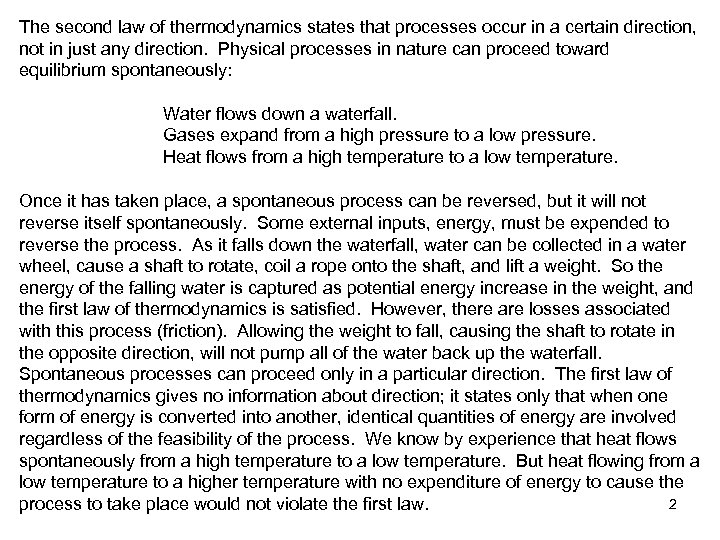The second law of thermodynamics states that processes occur in a certain direction, not in just any direction. Physical processes in nature can proceed toward equilibrium spontaneously: Water flows down a waterfall. Gases expand from a high pressure to a low pressure. Heat flows from a high temperature to a low temperature. Once it has taken place, a spontaneous process can be reversed, but it will not reverse itself spontaneously. Some external inputs, energy, must be expended to reverse the process. As it falls down the waterfall, water can be collected in a water wheel, cause a shaft to rotate, coil a rope onto the shaft, and lift a weight. So the energy of the falling water is captured as potential energy increase in the weight, and the first law of thermodynamics is satisfied. However, there are losses associated with this process (friction). Allowing the weight to fall, causing the shaft to rotate in the opposite direction, will not pump all of the water back up the waterfall. Spontaneous processes can proceed only in a particular direction. The first law of thermodynamics gives no information about direction; it states only that when one form of energy is converted into another, identical quantities of energy are involved regardless of the feasibility of the process. We know by experience that heat flows spontaneously from a high temperature to a low temperature. But heat flowing from a low temperature to a higher temperature with no expenditure of energy to cause the 2 process to take place would not violate the first law.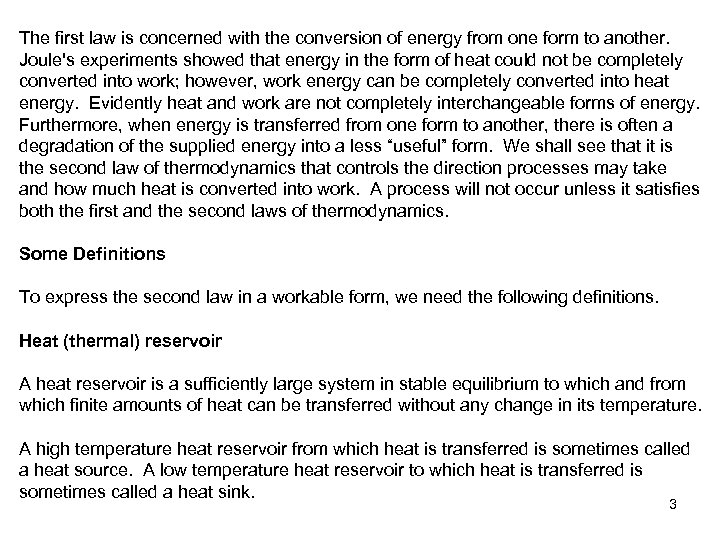The first law is concerned with the conversion of energy from one form to another. Joule's experiments showed that energy in the form of heat could not be completely converted into work; however, work energy can be completely converted into heat energy. Evidently heat and work are not completely interchangeable forms of energy. Furthermore, when energy is transferred from one form to another, there is often a degradation of the supplied energy into a less “useful” form. We shall see that it is the second law of thermodynamics that controls the direction processes may take and how much heat is converted into work. A process will not occur unless it satisfies both the first and the second laws of thermodynamics. Some Definitions To express the second law in a workable form, we need the following definitions. Heat (thermal) reservoir A heat reservoir is a sufficiently large system in stable equilibrium to which and from which finite amounts of heat can be transferred without any change in its temperature. A high temperature heat reservoir from which heat is transferred is sometimes called a heat source. A low temperature heat reservoir to which heat is transferred is sometimes called a heat sink. 3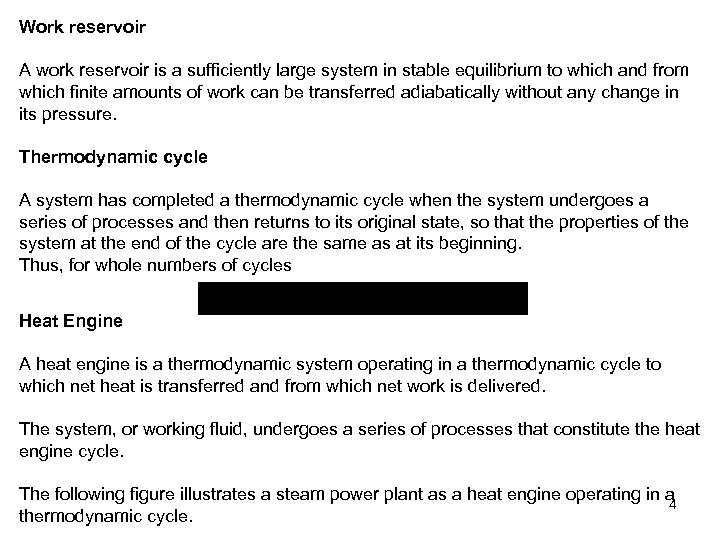Work reservoir A work reservoir is a sufficiently large system in stable equilibrium to which and from which finite amounts of work can be transferred adiabatically without any change in its pressure. Thermodynamic cycle A system has completed a thermodynamic cycle when the system undergoes a series of processes and then returns to its original state, so that the properties of the system at the end of the cycle are the same as at its beginning. Thus, for whole numbers of cycles Heat Engine A heat engine is a thermodynamic system operating in a thermodynamic cycle to which net heat is transferred and from which net work is delivered. The system, or working fluid, undergoes a series of processes that constitute the heat engine cycle. The following figure illustrates a steam power plant as a heat engine operating in a 4 thermodynamic cycle.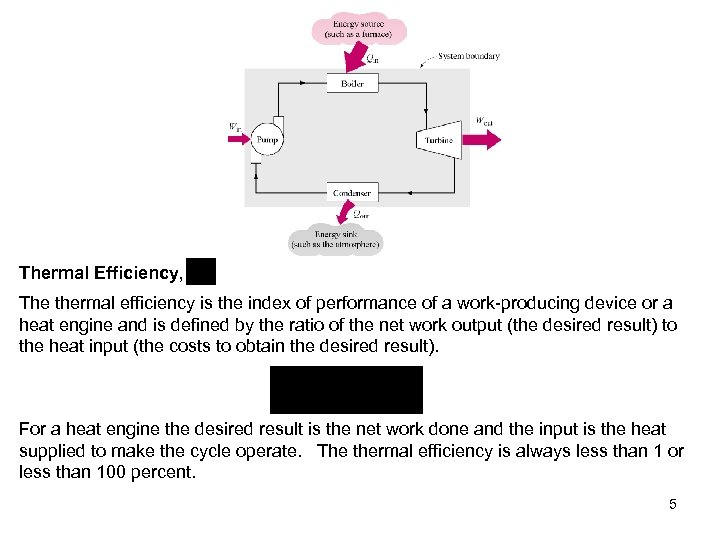Thermal Efficiency, The thermal efficiency is the index of performance of a work-producing device or a heat engine and is defined by the ratio of the net work output (the desired result) to the heat input (the costs to obtain the desired result). For a heat engine the desired result is the net work done and the input is the heat supplied to make the cycle operate. The thermal efficiency is always less than 1 or less than 100 percent. 5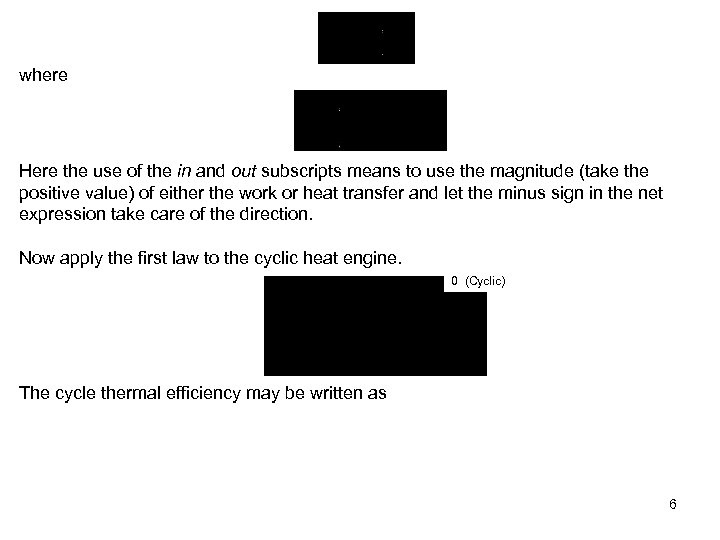where Here the use of the in and out subscripts means to use the magnitude (take the positive value) of either the work or heat transfer and let the minus sign in the net expression take care of the direction. Now apply the first law to the cyclic heat engine. 0 (Cyclic) The cycle thermal efficiency may be written as 6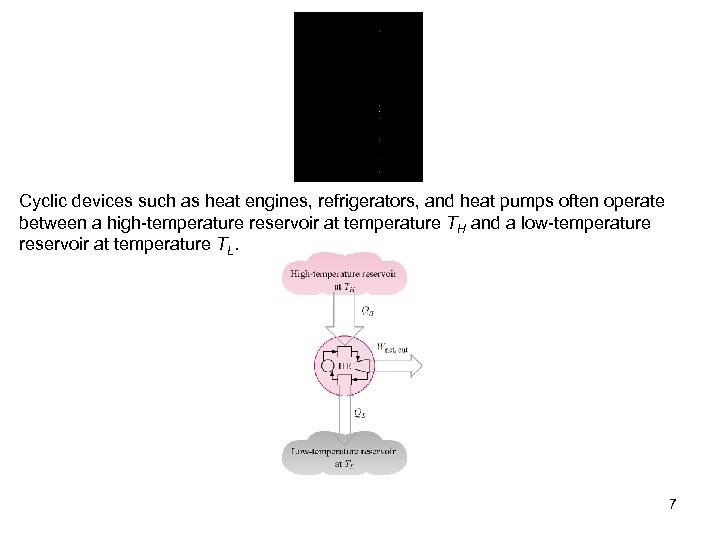Cyclic devices such as heat engines, refrigerators, and heat pumps often operate between a high-temperature reservoir at temperature TH and a low-temperature reservoir at temperature TL. 7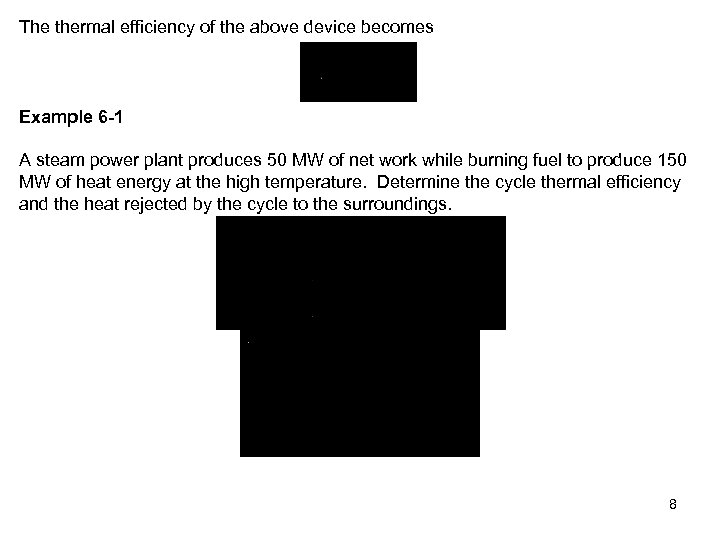The thermal efficiency of the above device becomes Example 6 -1 A steam power plant produces 50 MW of net work while burning fuel to produce 150 MW of heat energy at the high temperature. Determine the cycle thermal efficiency and the heat rejected by the cycle to the surroundings. 8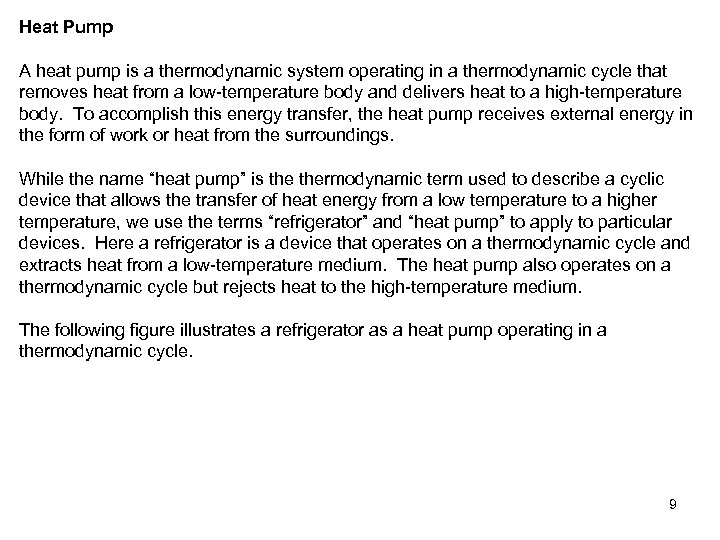Heat Pump A heat pump is a thermodynamic system operating in a thermodynamic cycle that removes heat from a low-temperature body and delivers heat to a high-temperature body. To accomplish this energy transfer, the heat pump receives external energy in the form of work or heat from the surroundings. While the name “heat pump” is thermodynamic term used to describe a cyclic device that allows the transfer of heat energy from a low temperature to a higher temperature, we use the terms “refrigerator” and “heat pump” to apply to particular devices. Here a refrigerator is a device that operates on a thermodynamic cycle and extracts heat from a low-temperature medium. The heat pump also operates on a thermodynamic cycle but rejects heat to the high-temperature medium. The following figure illustrates a refrigerator as a heat pump operating in a thermodynamic cycle. 9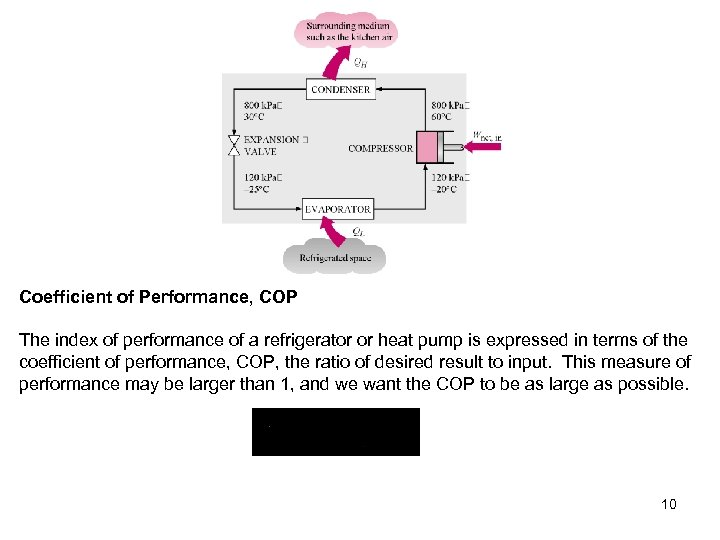Coefficient of Performance, COP The index of performance of a refrigerator or heat pump is expressed in terms of the coefficient of performance, COP, the ratio of desired result to input. This measure of performance may be larger than 1, and we want the COP to be as large as possible. 10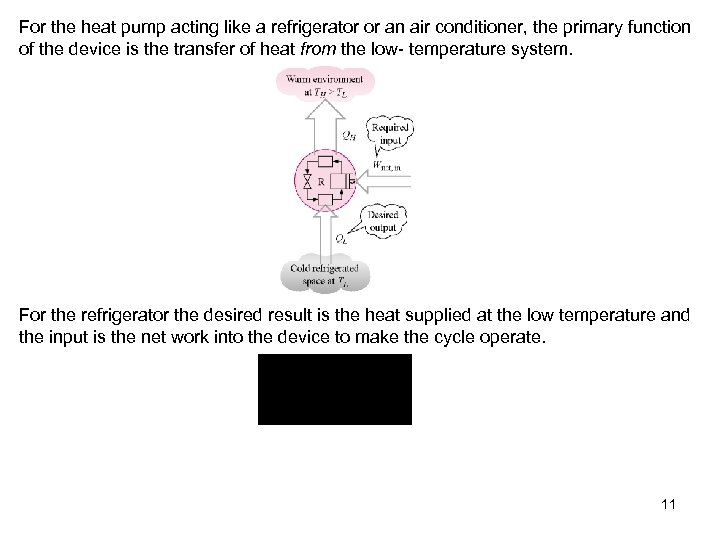For the heat pump acting like a refrigerator or an air conditioner, the primary function of the device is the transfer of heat from the low- temperature system. For the refrigerator the desired result is the heat supplied at the low temperature and the input is the net work into the device to make the cycle operate. 11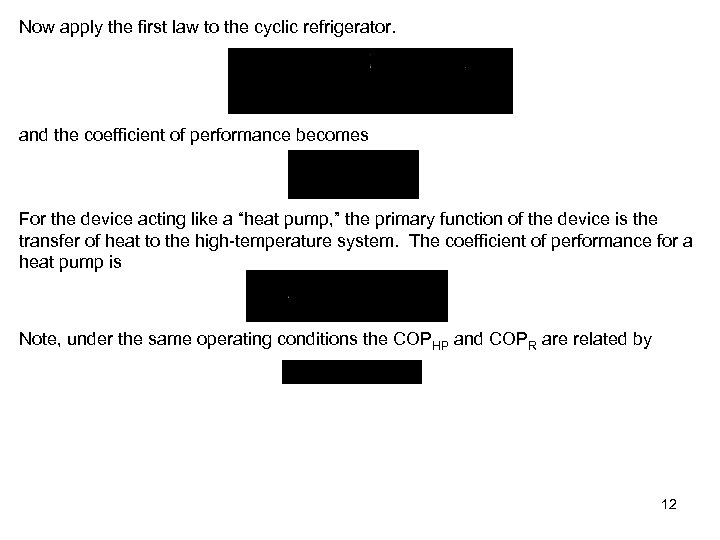Now apply the first law to the cyclic refrigerator. and the coefficient of performance becomes For the device acting like a “heat pump, ” the primary function of the device is the transfer of heat to the high-temperature system. The coefficient of performance for a heat pump is Note, under the same operating conditions the COPHP and COPR are related by 12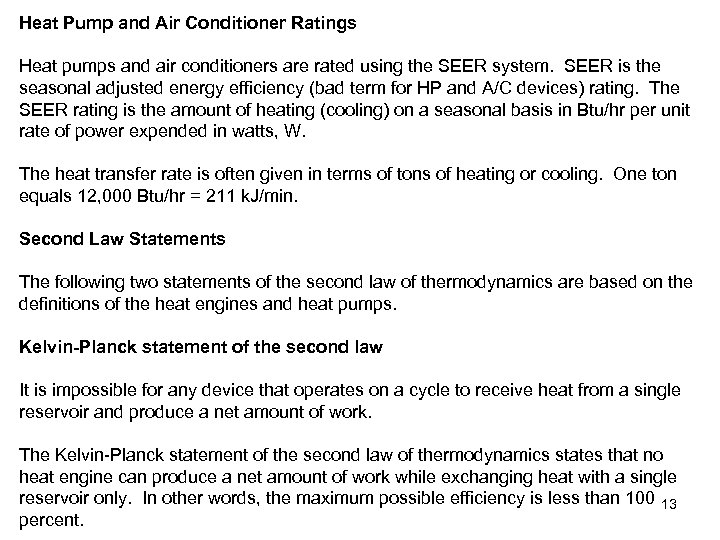Heat Pump and Air Conditioner Ratings Heat pumps and air conditioners are rated using the SEER system. SEER is the seasonal adjusted energy efficiency (bad term for HP and A/C devices) rating. The SEER rating is the amount of heating (cooling) on a seasonal basis in Btu/hr per unit rate of power expended in watts, W. The heat transfer rate is often given in terms of tons of heating or cooling. One ton equals 12, 000 Btu/hr = 211 k. J/min. Second Law Statements The following two statements of the second law of thermodynamics are based on the definitions of the heat engines and heat pumps. Kelvin-Planck statement of the second law It is impossible for any device that operates on a cycle to receive heat from a single reservoir and produce a net amount of work. The Kelvin-Planck statement of the second law of thermodynamics states that no heat engine can produce a net amount of work while exchanging heat with a single reservoir only. In other words, the maximum possible efficiency is less than 100 13 percent.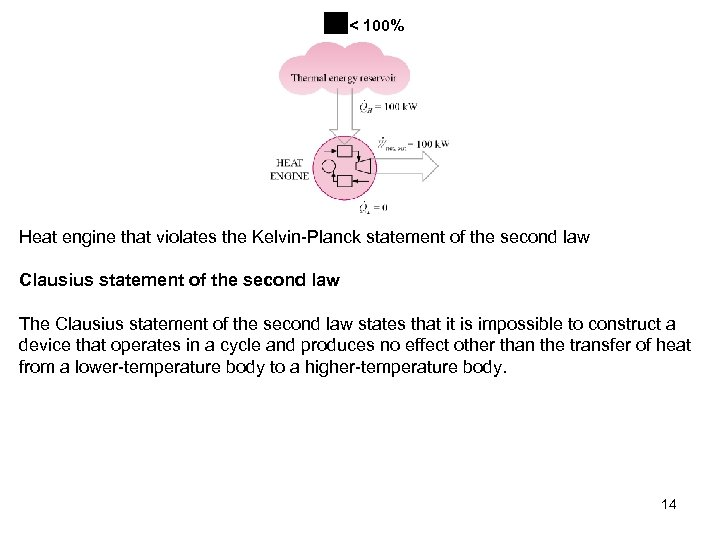< 100% Heat engine that violates the Kelvin-Planck statement of the second law Clausius statement of the second law The Clausius statement of the second law states that it is impossible to construct a device that operates in a cycle and produces no effect other than the transfer of heat from a lower-temperature body to a higher-temperature body. 14Heat pump that violates the Clausius statement of the second law Or energy from the surroundings in the form of work or heat has to be expended to force heat to flow from a low-temperature medium to a high-temperature medium. Thus, the COP of a refrigerator or heat pump must be less than infinity. 15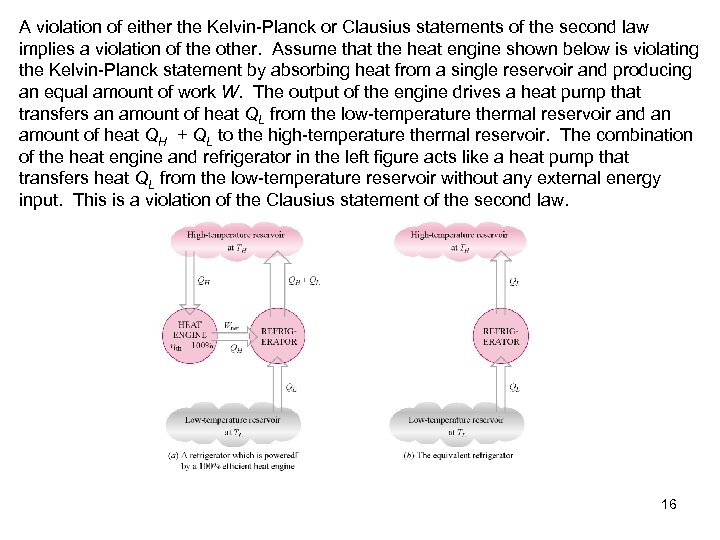A violation of either the Kelvin-Planck or Clausius statements of the second law implies a violation of the other. Assume that the heat engine shown below is violating the Kelvin-Planck statement by absorbing heat from a single reservoir and producing an equal amount of work W. The output of the engine drives a heat pump that transfers an amount of heat QL from the low-temperature thermal reservoir and an amount of heat QH + QL to the high-temperature thermal reservoir. The combination of the heat engine and refrigerator in the left figure acts like a heat pump that transfers heat QL from the low-temperature reservoir without any external energy input. This is a violation of the Clausius statement of the second law. 16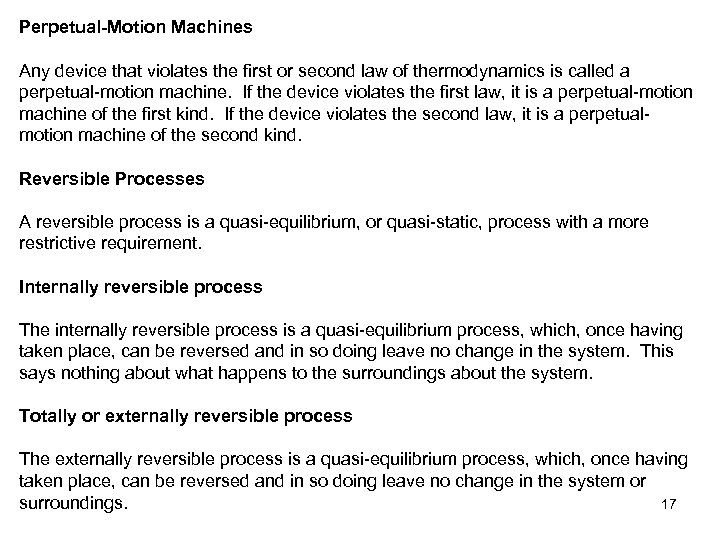Perpetual-Motion Machines Any device that violates the first or second law of thermodynamics is called a perpetual-motion machine. If the device violates the first law, it is a perpetual-motion machine of the first kind. If the device violates the second law, it is a perpetualmotion machine of the second kind. Reversible Processes A reversible process is a quasi-equilibrium, or quasi-static, process with a more restrictive requirement. Internally reversible process The internally reversible process is a quasi-equilibrium process, which, once having taken place, can be reversed and in so doing leave no change in the system. This says nothing about what happens to the surroundings about the system. Totally or externally reversible process The externally reversible process is a quasi-equilibrium process, which, once having taken place, can be reversed and in so doing leave no change in the system or surroundings. 17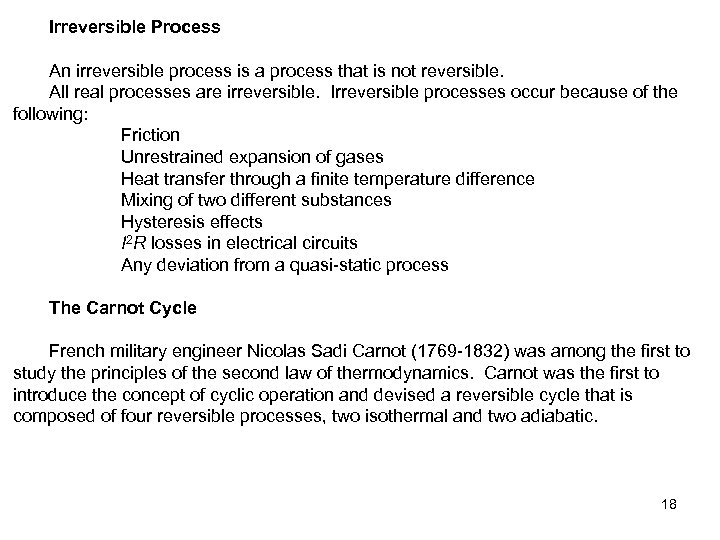Irreversible Process An irreversible process is a process that is not reversible. All real processes are irreversible. Irreversible processes occur because of the following: Friction Unrestrained expansion of gases Heat transfer through a finite temperature difference Mixing of two different substances Hysteresis effects I 2 R losses in electrical circuits Any deviation from a quasi-static process The Carnot Cycle French military engineer Nicolas Sadi Carnot (1769 -1832) was among the first to study the principles of the second law of thermodynamics. Carnot was the first to introduce the concept of cyclic operation and devised a reversible cycle that is composed of four reversible processes, two isothermal and two adiabatic. 18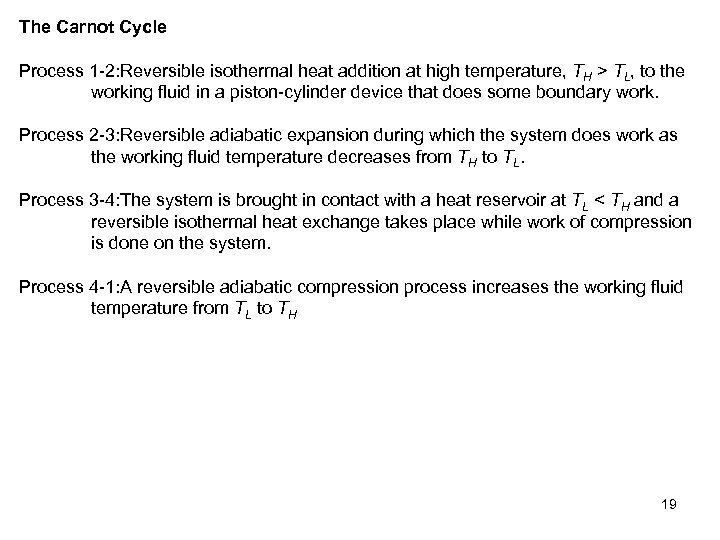The Carnot Cycle Process 1 -2: Reversible isothermal heat addition at high temperature, TH > TL, to the working fluid in a piston-cylinder device that does some boundary work. Process 2 -3: Reversible adiabatic expansion during which the system does work as the working fluid temperature decreases from TH to TL. Process 3 -4: The system is brought in contact with a heat reservoir at TL < TH and a reversible isothermal heat exchange takes place while work of compression is done on the system. Process 4 -1: A reversible adiabatic compression process increases the working fluid temperature from TL to TH 19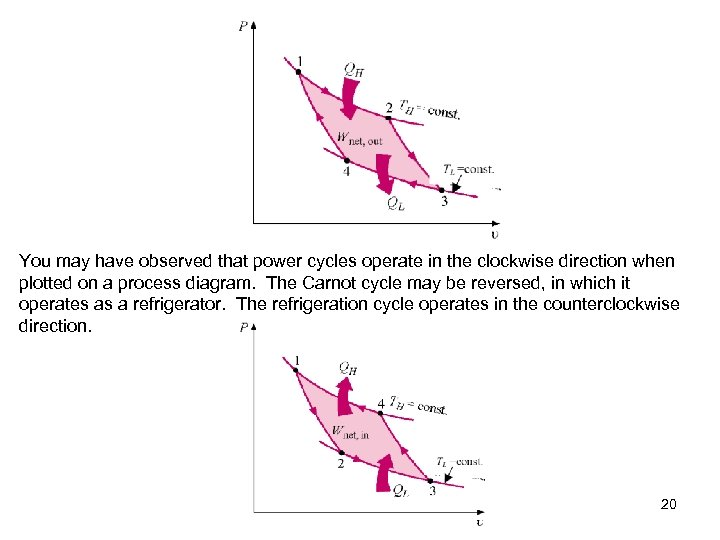You may have observed that power cycles operate in the clockwise direction when plotted on a process diagram. The Carnot cycle may be reversed, in which it operates as a refrigerator. The refrigeration cycle operates in the counterclockwise direction. 20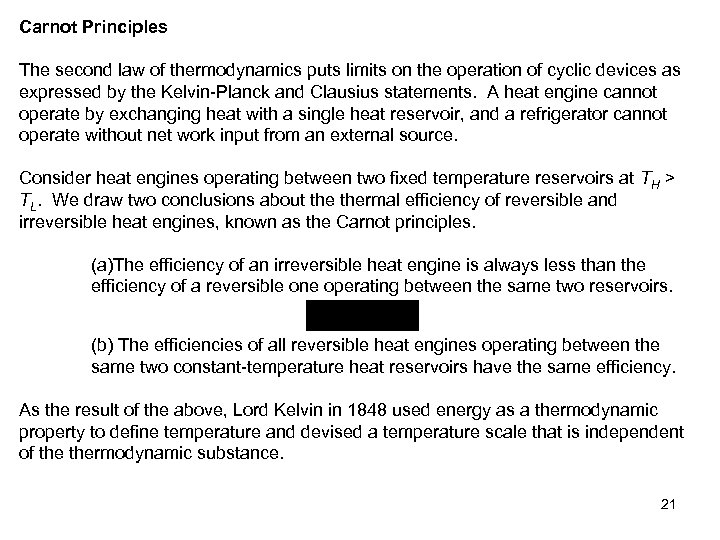Carnot Principles The second law of thermodynamics puts limits on the operation of cyclic devices as expressed by the Kelvin-Planck and Clausius statements. A heat engine cannot operate by exchanging heat with a single heat reservoir, and a refrigerator cannot operate without net work input from an external source. Consider heat engines operating between two fixed temperature reservoirs at TH > TL. We draw two conclusions about thermal efficiency of reversible and irreversible heat engines, known as the Carnot principles. (a)The efficiency of an irreversible heat engine is always less than the efficiency of a reversible one operating between the same two reservoirs. (b) The efficiencies of all reversible heat engines operating between the same two constant-temperature heat reservoirs have the same efficiency. As the result of the above, Lord Kelvin in 1848 used energy as a thermodynamic property to define temperature and devised a temperature scale that is independent of thermodynamic substance. 21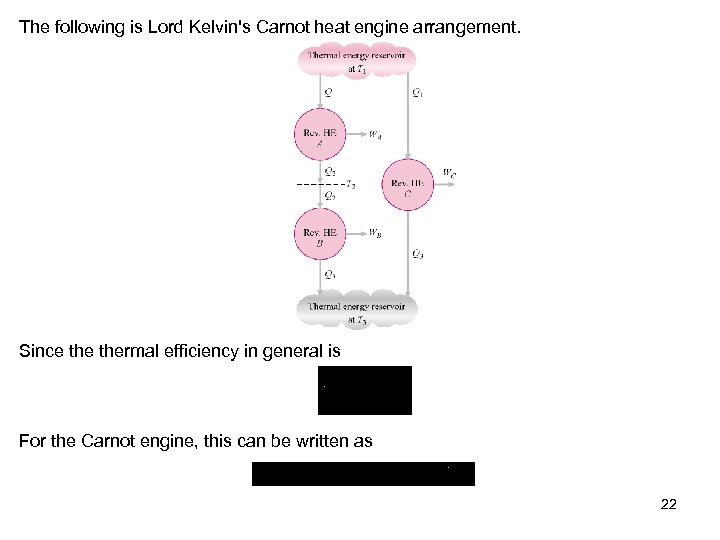The following is Lord Kelvin's Carnot heat engine arrangement. Since thermal efficiency in general is For the Carnot engine, this can be written as 22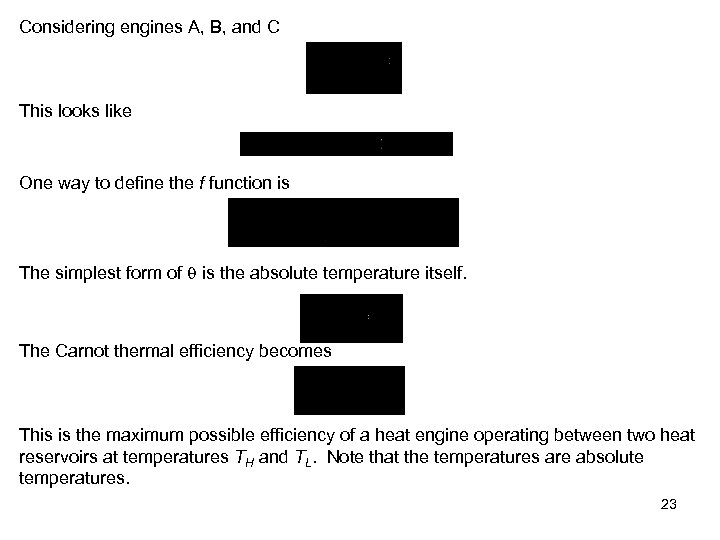Considering engines A, B, and C This looks like One way to define the f function is The simplest form of is the absolute temperature itself. The Carnot thermal efficiency becomes This is the maximum possible efficiency of a heat engine operating between two heat reservoirs at temperatures TH and TL. Note that the temperatures are absolute temperatures. 23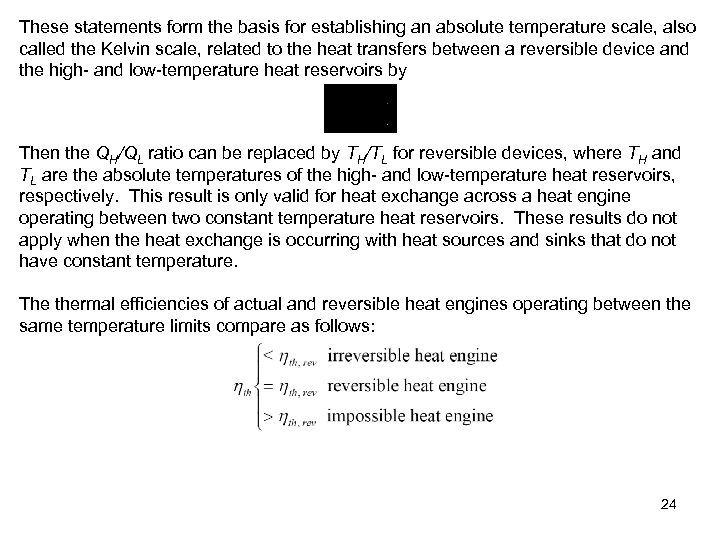These statements form the basis for establishing an absolute temperature scale, also called the Kelvin scale, related to the heat transfers between a reversible device and the high- and low-temperature heat reservoirs by Then the QH/QL ratio can be replaced by TH/TL for reversible devices, where TH and TL are the absolute temperatures of the high- and low-temperature heat reservoirs, respectively. This result is only valid for heat exchange across a heat engine operating between two constant temperature heat reservoirs. These results do not apply when the heat exchange is occurring with heat sources and sinks that do not have constant temperature. The thermal efficiencies of actual and reversible heat engines operating between the same temperature limits compare as follows: 24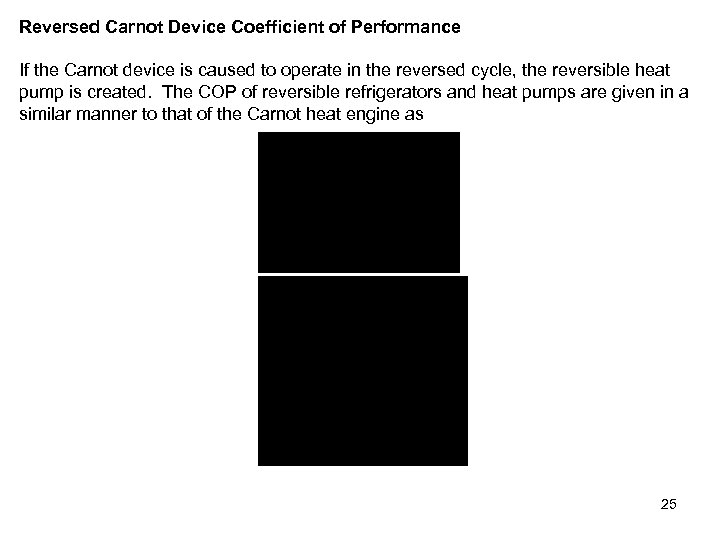Reversed Carnot Device Coefficient of Performance If the Carnot device is caused to operate in the reversed cycle, the reversible heat pump is created. The COP of reversible refrigerators and heat pumps are given in a similar manner to that of the Carnot heat engine as 25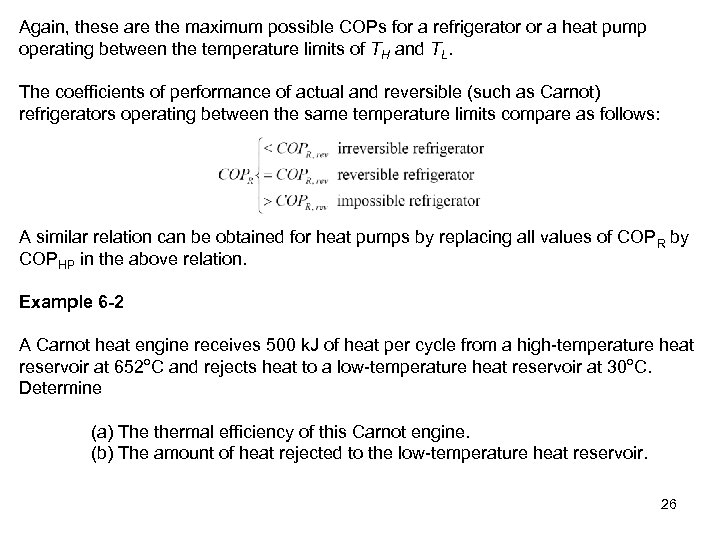Again, these are the maximum possible COPs for a refrigerator or a heat pump operating between the temperature limits of TH and TL. The coefficients of performance of actual and reversible (such as Carnot) refrigerators operating between the same temperature limits compare as follows: A similar relation can be obtained for heat pumps by replacing all values of COPR by COPHP in the above relation. Example 6 -2 A Carnot heat engine receives 500 k. J of heat per cycle from a high-temperature heat reservoir at 652 o. C and rejects heat to a low-temperature heat reservoir at 30 o. C. Determine (a) The thermal efficiency of this Carnot engine. (b) The amount of heat rejected to the low-temperature heat reservoir. 26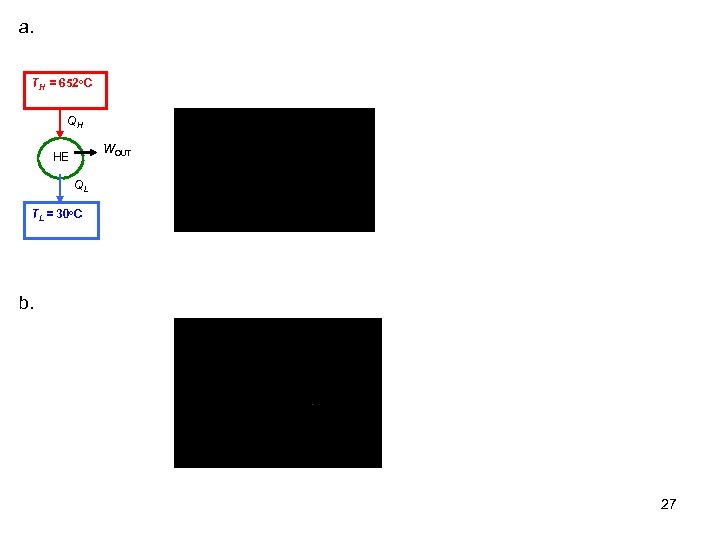a. TH = 652 o. C QH WOUT HE QL TL = 30 o. C b. 27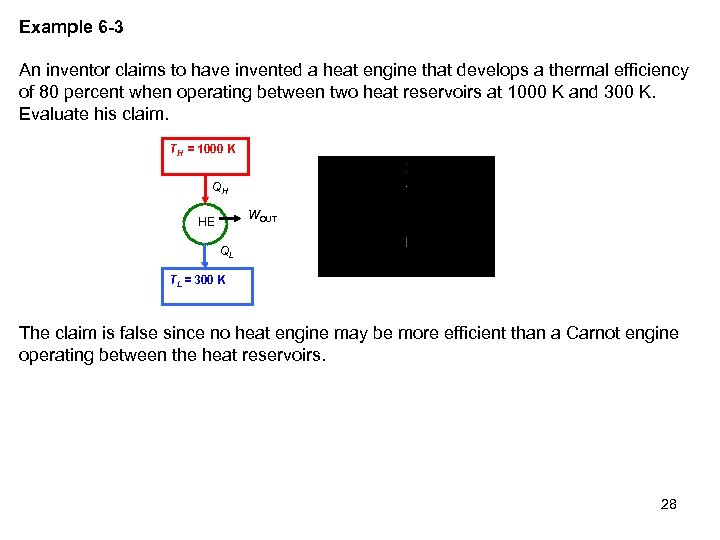Example 6 -3 An inventor claims to have invented a heat engine that develops a thermal efficiency of 80 percent when operating between two heat reservoirs at 1000 K and 300 K. Evaluate his claim. TH = 1000 K QH WOUT HE QL TL = 300 K The claim is false since no heat engine may be more efficient than a Carnot engine operating between the heat reservoirs. 28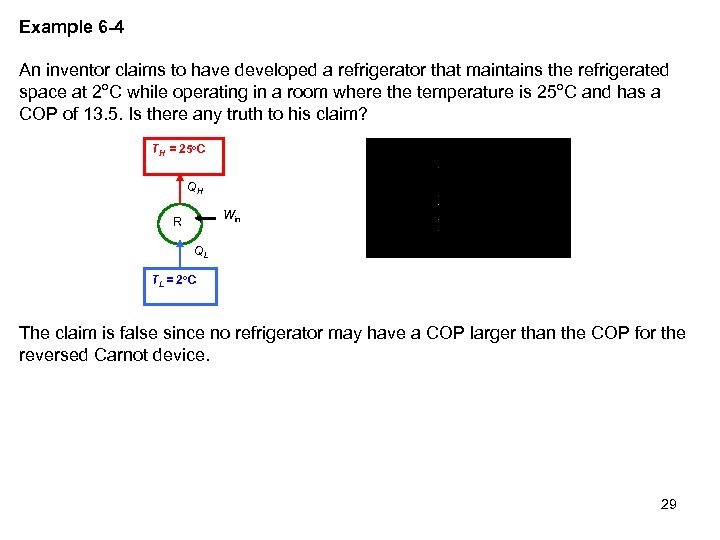Example 6 -4 An inventor claims to have developed a refrigerator that maintains the refrigerated space at 2 o. C while operating in a room where the temperature is 25 o. C and has a COP of 13. 5. Is there any truth to his claim? TH = 25 o. C QH Win R QL T L = 2 o C The claim is false since no refrigerator may have a COP larger than the COP for the reversed Carnot device. 29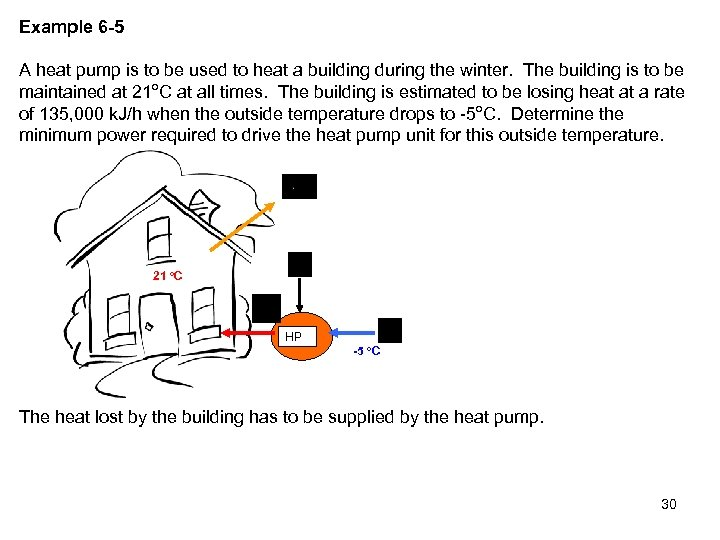Example 6 -5 A heat pump is to be used to heat a building during the winter. The building is to be maintained at 21 o. C at all times. The building is estimated to be losing heat at a rate of 135, 000 k. J/h when the outside temperature drops to -5 o. C. Determine the minimum power required to drive the heat pump unit for this outside temperature. 21 o. C HP -5 o. C The heat lost by the building has to be supplied by the heat pump. 30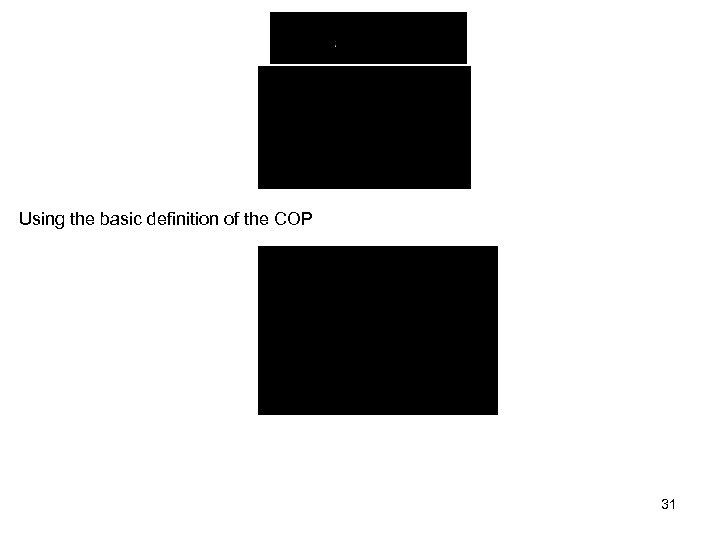Using the basic definition of the COP 31